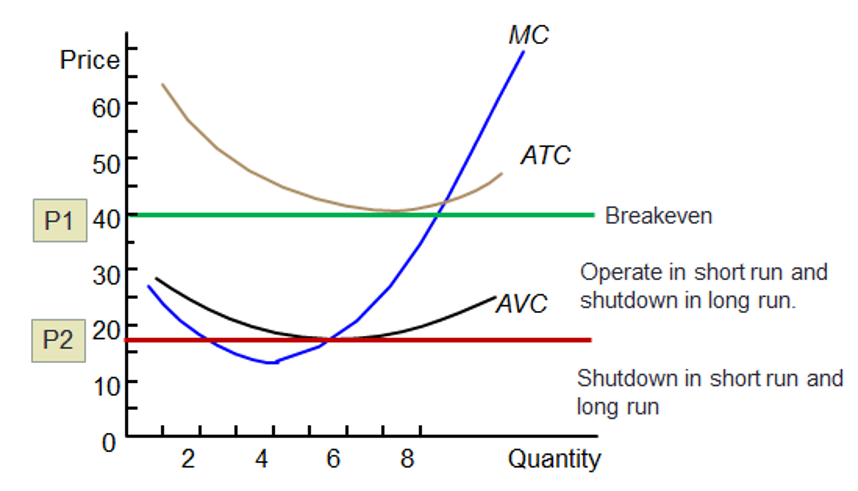# Supply Curve in Various Market Structures

### Perfect Competition

In perfect competition, the supply curve of an individual firm is positively sloped. The industry supply curve is also positively sloped, i.e., as the prices increase, the quantity supplied increases.

A firm’s short-run supply curve is the portion of the firm’s short-run marginal cost curve above average variable cost. This is because in the short-run, in order to be able to operate, the firm should recover its variable cost.

Remember that average variable cost curve is U-shaped. The average total cost curve is also U-shaped. The marginal cost curve is a J-shaped curve. In the graph below, the blue line from the point above AVC represents the short-run supply curve.The long-run market supply curve indicates the minimum price at which firms will supply various market output levels, given sufficient time both to adjust plant size and to enter or exit the industry In the long-run, it doesn’t make sense for the firm to operate below average total cost (ATC). Therefore, in the long run, the supply curve of a firm is the portion of the firm’s long-run marginal cost curve above average total cost.

### Monopolistic Competition

In a monopolistic market, there are instances where changes in demand curves do not produce a change in both price and quantity (P and Q). With a change in demand curve, a monopolist can either produce the same quantity but charge a different price or charge the same price but produce at a different quantity. Therefore, there is no one-to-one relationship between quantity and price—a monopolistic market has no supply curve.

### Oligopoly

An oligopoly firm also does not have a supply curve.

### Monopoly

Even a monopoly does not have a supply curve. A given quantity can correspond to more than one monopoly-optimal price. A shift in the demand curve may cause the monopoly optimal price to stay constant and the quantity to change or both price and quantity to change.